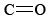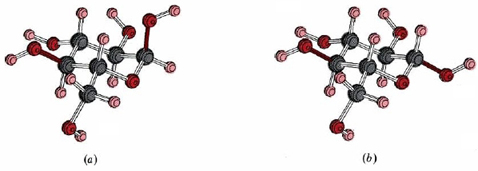$$\newcommand{\vecs}{\overset { \rightharpoonup} {\mathbf{#1}} }$$ $$\newcommand{\vecd}{\overset{-\!-\!\rightharpoonup}{\vphantom{a}\smash {#1}}}$$$$\newcommand{\id}{\mathrm{id}}$$ $$\newcommand{\Span}{\mathrm{span}}$$ $$\newcommand{\kernel}{\mathrm{null}\,}$$ $$\newcommand{\range}{\mathrm{range}\,}$$ $$\newcommand{\RealPart}{\mathrm{Re}}$$ $$\newcommand{\ImaginaryPart}{\mathrm{Im}}$$ $$\newcommand{\Argument}{\mathrm{Arg}}$$ $$\newcommand{\norm}{\| #1 \|}$$ $$\newcommand{\inner}{\langle #1, #2 \rangle}$$ $$\newcommand{\Span}{\mathrm{span}}$$ $$\newcommand{\id}{\mathrm{id}}$$ $$\newcommand{\Span}{\mathrm{span}}$$ $$\newcommand{\kernel}{\mathrm{null}\,}$$ $$\newcommand{\range}{\mathrm{range}\,}$$ $$\newcommand{\RealPart}{\mathrm{Re}}$$ $$\newcommand{\ImaginaryPart}{\mathrm{Im}}$$ $$\newcommand{\Argument}{\mathrm{Arg}}$$ $$\newcommand{\norm}{\| #1 \|}$$ $$\newcommand{\inner}{\langle #1, #2 \rangle}$$ $$\newcommand{\Span}{\mathrm{span}}$$Figure $$\PageIndex{1}$$ we have indicated the structure of the sugars in linear or chain form, but sugars usually occur in one of several ring or cyclic structures. We will only consider two of these, the α and β form of glucose. Because of the flexibility of the carbon chain, the linear form of glucose can easily adopt a conformation in which carbon atom 1 lies adjacent to the oxygen atom on carbon atom 5. When this happens, a proton can be transferred and a carbon-oxygen bond formed:Figure $$\PageIndex{1}$$ The linear form of some important monosaccharides: (a) ribose; (b) fructose; (c) mannose; (d) glucose.
A careful consideration of the geometry of this structure reveals that not one but two cyclic structures are possible. These are called α- and β-glucose and are shown in Figure $$\PageIndex{2}$$. In the β form the C—O bond on carbon atom 1 (shown in dark color) is parallel to the C—O bond on carbon atom 4, while in the α form these two bonds are at an angle of 180° – 109.5° = 70.5°. This geometric difference may seem relatively trivial, but it turns out to be important when glucose molecules are used as building blocks to form larger entities.Figure $$\PageIndex{2}$$ (a) α-glucose; (b) β-glucose. Note how the C—O bonds protruding from the left- and right-hand sides of the molecule (indicated in color) are parallel to each other in the β form but not in the α form.